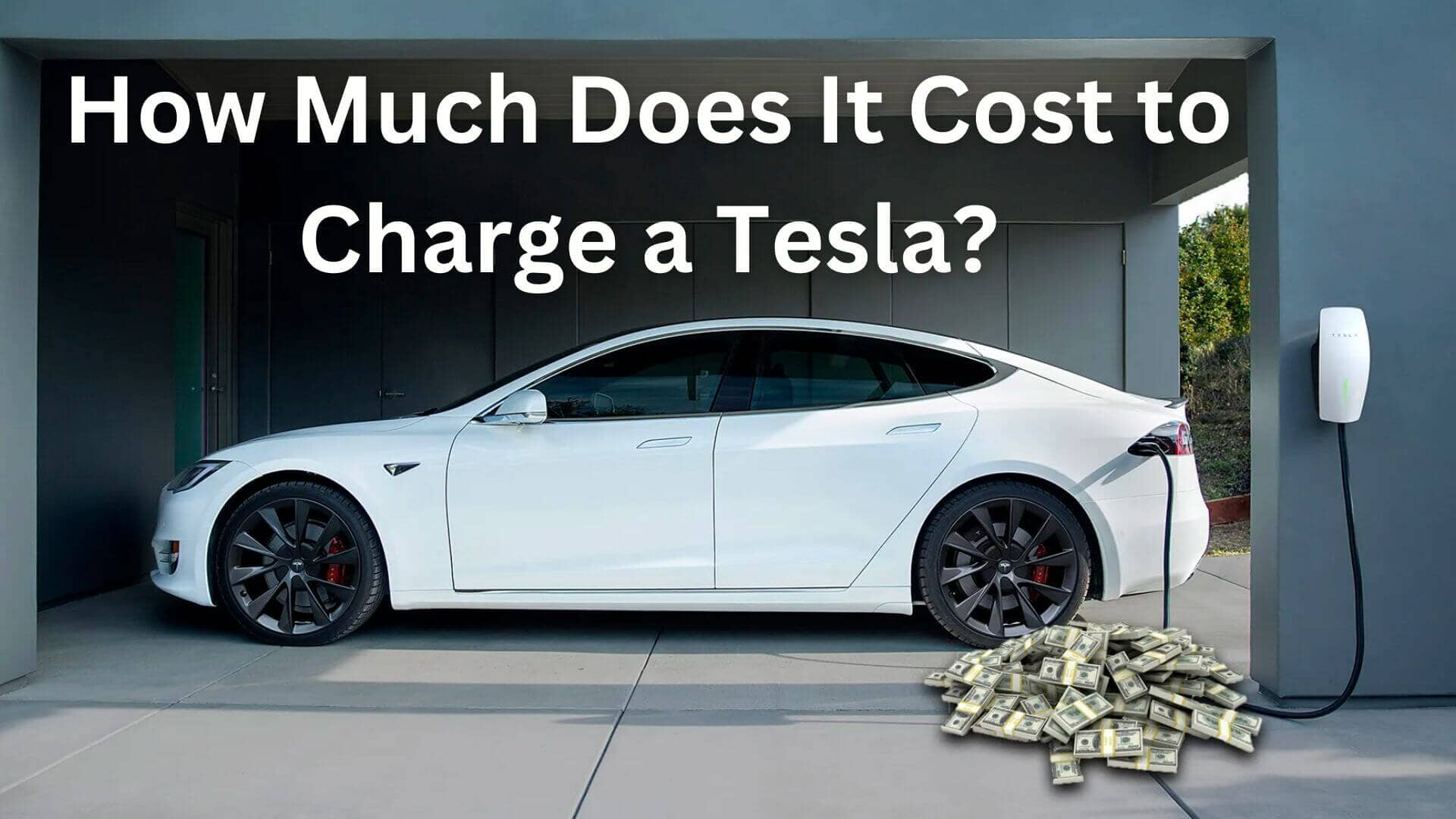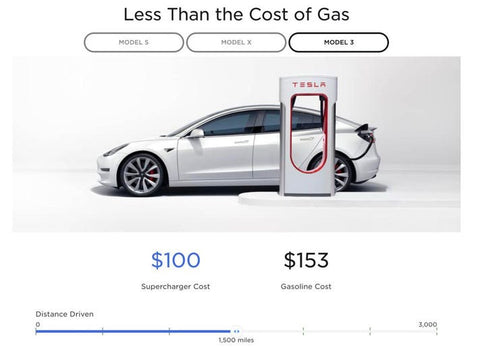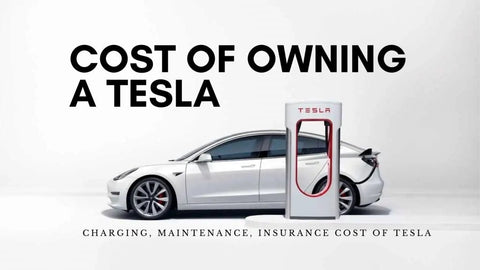# How Much Does It Cost to Charge a Tesla? A Comprehensive Guide

Are you contemplating a Tesla but wondering about charging costs? It's much cheaper than petroleum. Tesla charging costs vary by model and technique. A home battery charges a Model 3 for \$11.47 and a Model Y for \$15.36. Tesla's Supercharger stations cost \$0.28 per kWh, so a full charge costs \$20 to \$30, based on the model. Let's examine the factors that influence Tesla charging costs and how to calculate them.

## IntroductionAre you thinking about purchasing a Tesla but are inquisitive about how much it will cost you to charge it? The good news is that it is significantly less expensive than maintaining a gas-powered vehicle. The price of charging a Tesla varies widely from model to model and also depends on the charging technique that is chosen. When using a home charger, it will typically cost around \$11.47 to completely charge a Model 3, and it will cost around \$15.36 for a Model Y.

Supercharger stations, which are Tesla's proprietary charging stations, cost an average of \$0.28 per kWh, which means that it costs approximately \$20 to \$30 for a full charge, based on the model. Supercharger stations can be found in over 70 countries around the world. Let's get into the nitty-gritty of how much it costs to charge a Tesla, including the variables that influence the price as well as the formula for determining it.

Tesla has revolutionized the auto industry with its electric cars, but one question that often comes up for potential buyers is: how much does it cost to charge a Tesla? In this comprehensive guide, we'll explore the various factors that affect the cost of charging a Tesla, including how many kWh are needed to charge a Model 3 or Model Y, the cost of using Tesla Superchargers, and the cost of installing a Tesla charging station at home. Read on to discover everything you need to know about the cost of charging a Tesla.

## How Many kWh to Charge a Tesla?

The number of kWh required to charge a Tesla depends on the battery capacity of the car and the charging rate. As a general rule, a Tesla Model 3 or Model Y with a Long Range battery pack requires approximately 75 kWh to charge from empty to full, while a Standard Range model requires around 55 kWh.

## How Much Does it Cost to Charge a Tesla Model 3?

The cost of charging a Tesla Model 3 varies depending on the electricity rates in your area and the charging method used. Using a Level 1 charger at home can cost around \$11 for a full charge, while using a Level 2 charger can cost around \$6.50 for a full charge. Using a Tesla Supercharger can cost around \$22 for a full charge.## How Much Does it Cost to Charge a Tesla Model Y?

The cost of charging a Tesla Model Y is similar to that of a Model 3, with the cost varying depending on the charging method and electricity rates. Using a Level 1 charger at home can cost around \$14 for a full charge, while using a Level 2 charger can cost around \$8 for a full charge. Using a Tesla Supercharger can cost around \$28 for a full charge.

## Do You Have to Pay to Charge a Tesla?

Charging a Tesla at home using a Level 1 or Level 2 charger requires payment for the electricity used, but there are no additional fees or subscriptions required. Using a Tesla Supercharger, on the other hand, does require payment, with the cost per kWh varying by location.

## Tesla Supercharger Cost Per kWh

The cost per kWh for using a Tesla Supercharger varies by location and can range from around \$0.24 to \$0.32 per kWh. Using a Supercharger to charge a Tesla can be more expensive than charging at home, but it's a convenient option for long-distance travel.

## How Much Do Tesla Charging Stations Cost?

The cost of installing a Tesla charging station at home can vary depending on the electrical work required and the type of charging station chosen. A basic Level 2 charging station can cost around \$500, while a more advanced station with additional features can cost up to \$1,200.

## Cost to Charge a Tesla: Factors to ConsiderFactors that can affect the cost to charge a Tesla include the battery capacity of the car, the charging rate, the electricity rates in your area, and the charging method used. To calculate the cost of charging your Tesla, you can use online calculators or consult with your electricity provider to determine the cost per kWh in your area.

Here's a Youtube video on 'How Much does it cost to charge a Tesla'.